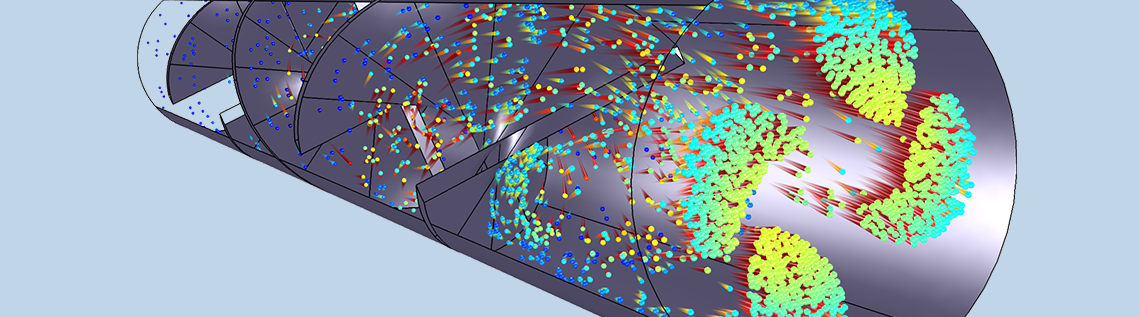# 借助仿真 App 评估静态混合器的性能

2016年 7月 8日

### 层流静态粒子混合器设计器 App 的设计基础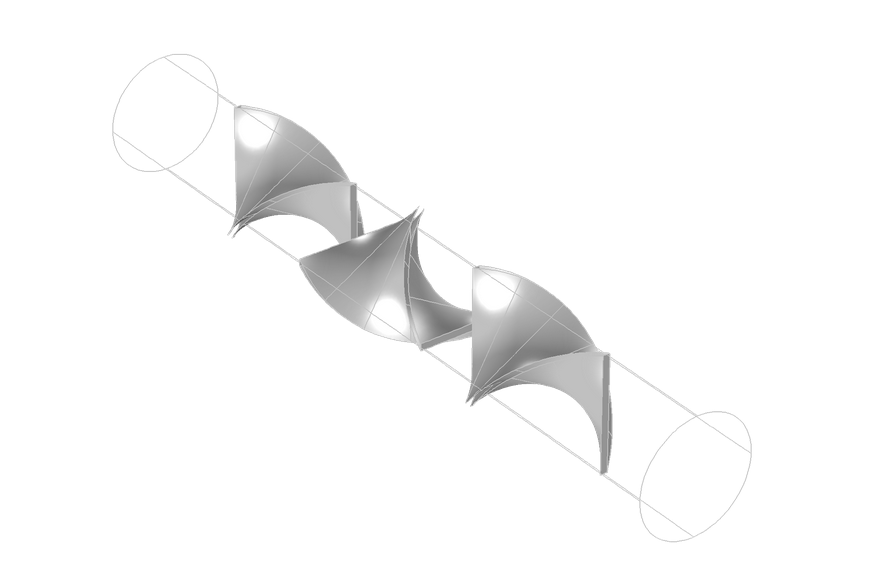### 用于研究静态混合器性能的 App“层流静态粒子混合器设计器”的用户界面（UI）截图。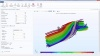(1)

\frac{d}{dt}\left(m_p\frac{d\mathbf{q}}{dt}\right) = \mathbf{F}_t

(2)

\begin{aligned}
\mathbf{F}_D &= \frac{1}{\tau_p} m_p \left(\mathbf{u}-\mathbf{v}\right)\\
\tau_p &= \frac{\rho_p d_p^2}{18 \mu}
\end{aligned}

• \mathbf{v}（国际单位：m/s）表示粒子速度
• \mathbf{u}（国际单位：m/s）表示流体速度
• d_p（国际单位：m）表示粒子直径
• \rho_p（国际单位：kg/m^3）表示粒子密度
• \mu（国际单位：kg/(m\cdot s)）表示粒子动力粘度

Stokes 曳力定律适用于相对雷诺数远小于 1 的粒子；也就是，

(3)

\textrm{Re}_r = \frac{\rho \parallel {\mathbf{u}-\mathbf{v}}\parallel d_p}{\mu} \ll 1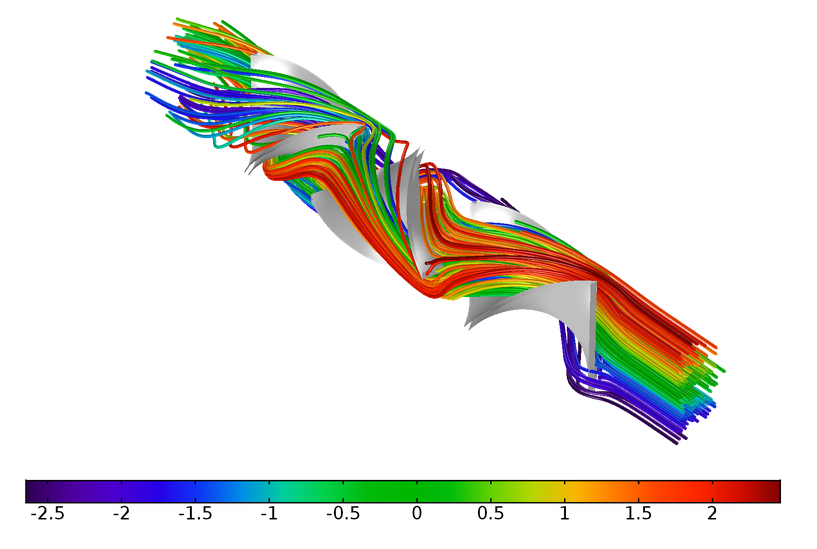### 借助 App 开发器量化静态混合器的性能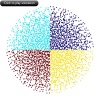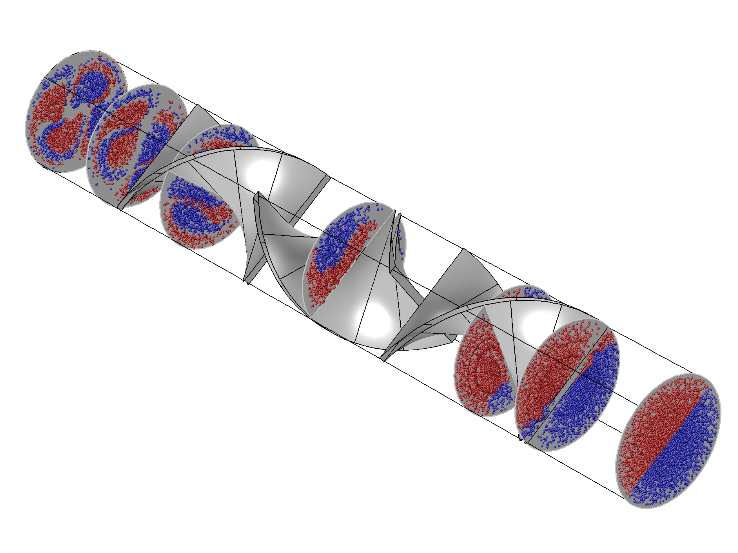(4)

D=\frac{\sigma^2}{\mu}

r_i = \sqrt{\frac{i}{N_r}} \hspace{1cm} \textrm{for } i=1,2,3\ldots N_r-1

\phi_j = \frac{2\pi j}{N_{\phi}} \hspace{1cm} \textrm{for } j=0,1,2\ldots N_{\phi}-1

\bar{x}=\frac{1}{N_q}\sum_{i=1}^{N_q} x_i

\sigma = \frac{1}{N_q}\sum_{i=1}^{N_q} (x_i-\bar{x})^2

/*
* p_computeIndexOfDispersion
* This method computes the index of dispersion at the outlet.
* The method is called in p_initApplication and in m_compute.
*/

// Get the x- and z-coordinates of the particles at the outlet
// and store them in matrices qx and qz, respectively.
model.result().numerical().create("par1", "Particle");
model.result().numerical("par1").set("solnum", new String[]{"14"});
model.result().numerical("par1").set("expr", "qx");
model.result().numerical("par1").set("unit", "m");
double[][] qx = model.result().numerical("par1").getReal();
model.result().numerical("par1").set("expr", "qz");
model.result().numerical("par1").set("unit", "m");
model.result().numerical("par1").set("solnum", new String[]{"14"});
double[][] qz = model.result().numerical("par1").getReal();

// Use the "at" operator to get the initial x-coordinates of all particles
// and store them in matrix qx0.
model.result().numerical("par1").set("expr", "at(0,qx)");
model.result().numerical("par1").set("unit", "m");
model.result().numerical("par1").set("solnum", new String[]{"14"});
double[][] qx0 = model.result().numerical("par1").getReal();

// The Particle Evaluation is no longer needed.
model.result().numerical().remove("par1");

double Ra = model.param().evaluate("Ra"); // Radius of the outlet
int Np = qx.length;                      // Number of particles
int Nr = nbrR;                            // Number of subdivisions in the radial direction
int Nphi = nbrPhi;                        // Number of subdivisions per quadrant in the azimuthal direction
double deltaPhi = Math.PI/(2*Nphi);       // Angular width of each quadrat
int index = 0;
int ir = 0;
int iphi = 0;
int[] x = new int[nbrQuad]; // Array to store number of points per quadrat

// Begin loop over all particles
for (int i = 0; i < Np; ++i) {
// Determine which quadrat each particle is in.
ir = (int) Math.floor((Math.pow(qx[i], 2)+Math.pow(qz[i], 2))*Nr/Math.pow(Ra, 2));
iphi = (int) Math.floor(Math.atan2(qz[i], qx[i])*Math.signum(qz[i])/deltaPhi);
if (Math.signum(qz[i]) < 0) {
iphi = (int) Math.floor((2*Math.PI-Math.atan2(qz[i], qx[i])*Math.signum(qz[i]))/deltaPhi);
}
index = 4*Nphi*ir+iphi;
// Consider only half of the particles when evaluating mixer performance.
if (qx0[i] < 0) {
x[index] = x[index]+1;
}
}
// compute the mean
double sum = 0;
for (int i = <0; i < nbrQuad; ++i) {
sum += x[i];
}
// compute the variance
sum = 0;
for (int i = 0; i < nbrQuad; ++i) {
sum += Math.pow(x[i]-xmean, 2);
}
indexOfDispersion = sum/xmean;


### 指导 App 开发的实用资源

• 自己动手尝试链接中的演示 App：层流静态粒子混合器设计器
• 如您希望了解更多关于提升仿真 App 结构和设计的方法，请阅读下列博客文章：